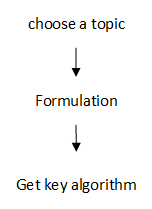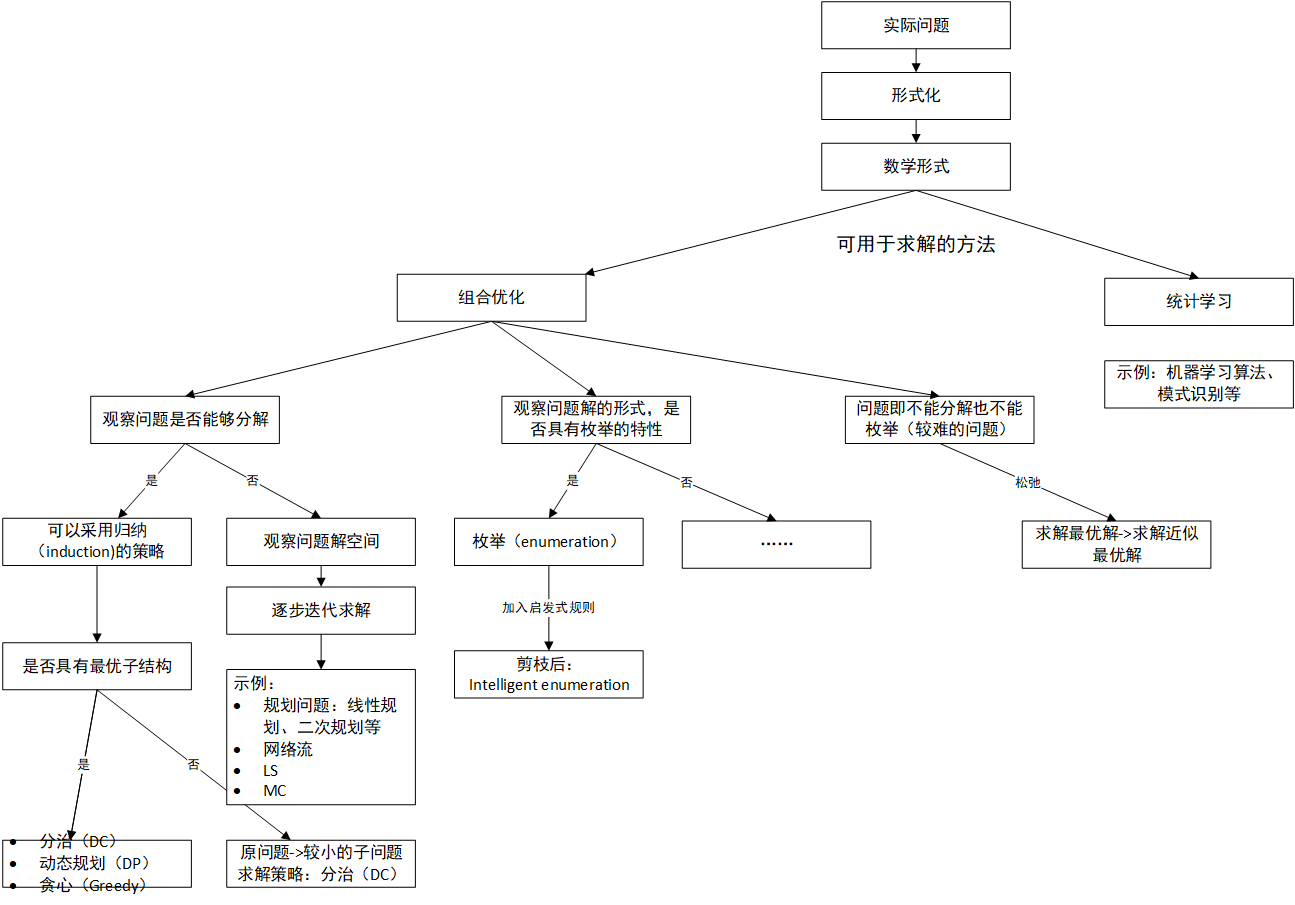# 算法小结(一)-问题求解背后的思考方式

2023年做了一次网站迁移，有价值的旧文重发

## 概述

• 找到需要解决的实际问题
• 将实际问题形式化为数学形式
• 找到求解问题的算法• 组合优化可以求解的问题，这类问题有着悠久的历史，经典的TSP、传统的排序算法都是属于这一类算法。为了描述方便，这类问题在文章中简记为C.U.问题(combination and optimization)
• 统计学习可以求解的问题，这类问题通常可以用机器学习、模式识别这样的方式求解。为了描述方便，这类问题在文章中简记为STAT问题

## C.U.问题

• 观察问题是否可以分解，可以分解就尝试采用归纳（induction）的策略
• 问题不能分解，观察问题的解空间，是否可以尝试逐步迭代求解(improvement)
• 观察问题解的形式，可以发现问题是否具有枚举的特性，如果具备这样的性质，可以尝试采用枚举（enumeration）的思想求解问题

### 问题是否可以分解

1. Formulation：

Input： 两个n位数a和b(a>=b) Output: gcd(a,b)

1. 分析过程
a和b非常抽象，我们给定其具体的值来观察分析，求解gcd(101,1949)

> 对例子进行观察，可以得出
> gcd(101,1949)=gcd(101,1949 mod 101)=gcd(101,30)
> 这样就将原问题reduce to a smaller problem，由此可以得到下述reduce的过程
> gcd(101,1949)=gcd(101,1949 mod 101)=gcd(101,30)
> gcd(101,30)=gcd(101 mod 30,30)=gcd(11,30)
> gcd(11,30)=gcd(11,30 mod 11)=gcd(11,8)
> gcd(11,8)=gcd(11 mod 8,8)=gcd(3,8)
> gcd(3,8)=gcd(3,8 mod 3)=gcd(3,2)
> gcd(3,2)=gcd(3 mod 2,2)=gcd(1,2)
> gcd(1,2)=gcd(1,2 mod 1)=gcd(1,1)
> gcd(1,1)=gcd(1,1 mod 1)=gcd(1,0)=1




function gcd(a, b)
while b ≠ 0
t ← b
b ← a mod b
a ← t
return a


1. 如何判断一个问题是否可以reduce？ 关键在于两部分：reduce得到的子问题是否易解子问题的解是否容易合并为原问题的解。是否reduce可以用一个很朴素的方式来判断： 问题规模为n时不易解，则先考虑最简单的情况：当$n=1$时的解，再考虑当$n=2$时，$\cdots$。最后观察是否可以通过这样的过程归纳出规模为n问题的求解算法。reduce通常有两种形式，这里以排序n个数为例：

• 增量式：先一个数排序，再增加一个数，$2$个数排序，直到$n$个数排序，这是插入排序的思想
• 分治式：$n$个数先分成两半，$n/2$规模的问题再分成两半得到$n/4$规模的问题，直至子问题易求解，这是归并排序的思想

增量式的reduce策略往往对应动态规划、贪心的求解过程；分治式的reduce策略往往对应分治算法的求解过程。

注意reduce得到的子问题和原问题的形式是类似的，即子问题可以用类似的方式求解

2. 问题可以reduce，如何得到原问题的解

• 若问题可以reduce，则可以用分治求解
• 若问题可以reduce、具有最优子结构，则可以尝试用动态规划求解
• 若问题可以reduce、具有最优子结构、满足贪心选择的性质，可以尝试用贪心求解 贪心选择性质：可以由局部最优解得到全局最优解，即在算法的每一步都可以容易地得到局部最优解，由于具有最优子结构的性质，则最后得到的解一定是全局最优的

• 数组
• 分治式：$n$维数组分解为两个$n/2$的数组，然后继续分解直至子问题易解
• 增量式：考虑小规模问题：$n=1, n=2, \cdots$
• 矩阵
• 分治式：$n\times m$的矩阵，四等分，得到4个$\frac{n}{2}\times \frac{m}{2}$的小矩阵，重复至子问题易解
• 增量式：$(i+1,j+1)$规模的子问题，到$(i+1,j), (i,j+1)$规模的子问题
• 树的子树往往就代表着子问题

• 从“smaller subproblems"中归纳出原问题的求解算法
• 算法的正确性往往可以通过数学归纳法证明

### 观察问题的解空间

• 节点：一个节点对于问题的一个complete solution
• 边：两个节点之间存在边，代表这两个节点是邻居（neighboors），即可以通过“简单”操作从一个complete solution转换到另一个complete solution。如果两个solution在解空间中是邻居关系，则通常这两个解的差别并不会太大。• 给定初始解是s：a->b->c->d->e->a (路程：25)
• 这时开始寻找初始解的邻居，上面已经介绍，邻居与现有解的差异应该尽可能小（即需要转到的解与现有解的差异应当很小，原因会在线性规划部分进行详述）；定义解之间不同边的个数为差异程度d，显然由于条件限制，d最小为2
• 由此可以得到一个基于s的，improved的解s’：a->b->c->e->d->a (路程：23)